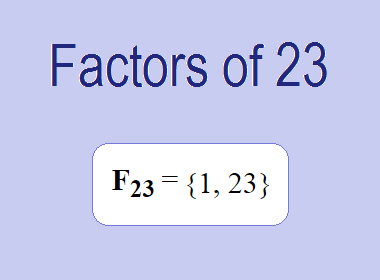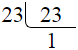# Factors of 23The factors of 23 are 23 are 1 and 23 i.e. F23 = {1, 23}. The factors of 23 are all the numbers that can divide 23 without leaving a remainder. 23 is a prime number, so it is divisible by 1 and 23 only.

We can check if these numbers are factors of 23 by dividing 23 by each of them. If the result is a whole number, then the number is a factor of 23. Let's do this for each of the numbers listed above:

·        1 is a factor of 23 because 23 divided by 1 is 23.

·        23 is a factor of 23 because 23 divided by 23 is 1.

## Properties of the Factors of 23

The factors of 23 have some interesting properties. One of the properties is that the sum of the factors of 23 is equal to 24. We can see this by adding all the factors of 23 together:

1 + 23 = 24

Another property of the factors of 23 is that the only prime factor of 23 is 23 itself.

********************

********************

## Applications of the Factors of 23

The factors of 23 have several applications in mathematics. One of the applications is in finding the highest common factor (HCF) of two or more numbers. The HCF is the largest factor that two or more numbers have in common. For example, to find the HCF of 23 and 46, we need to find the factors of both numbers and identify the largest factor they have in common. The factors of 23 are 1, and 23. The factors of 46 are 1, 2, 23, and 46. The largest factor that they have in common is 23. Therefore, the HCF of 23 and 46 is 23.

Another application of the factors of 23 is in prime factorization. Prime factorization is the process of expressing a number as the product of its prime factors. The prime factor of 23 is 23 since it is only the prime number that can divide 23 without leaving a remainder. Therefore, we can express 23 as:

23 = 23

We can do prime factorization by division method as given below,23 = 23

Since 23 is a prime number, there is no factor tree of 23.

## Conclusion

The factors of 23 are the numbers that can divide 23 without leaving a remainder. The factors of 23 are 1, and 23. The factors of 23 have some interesting properties, such as having a sum of 24. The factors of 23 have several applications in mathematics, such as finding the highest common factor and prime factorization.